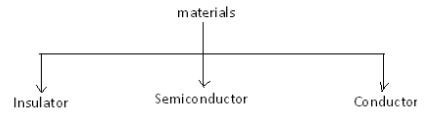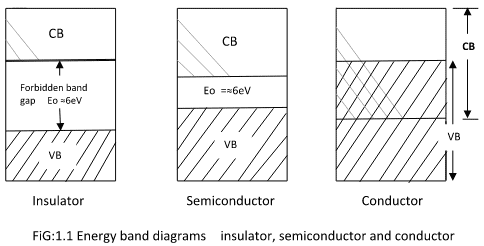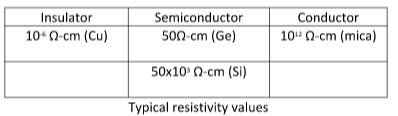Courses

# Pn Junction Diode Electrical Engineering (EE) Notes | EduRev

## Electrical Engineering (EE) : Pn Junction Diode Electrical Engineering (EE) Notes | EduRev

The document Pn Junction Diode Electrical Engineering (EE) Notes | EduRev is a part of the Electrical Engineering (EE) Course Analog Electronics.
All you need of Electrical Engineering (EE) at this link: Electrical Engineering (EE)

INTRODUCTION

Based on the electrical conductivity all the materials in nature are classified as insulators,
semiconductors, and conductors.Insulator: An insulator is a material that offers a very low level (or negligible) of conductivity when a voltage is applied. Eg: Paper, Mica, glass, quartz. Resistivity level of an insulator is of the order of 1010 to 1012 Ω-cm. The energy band structure of an insulator is shown in the fig.1.1. Band structure of material defines the band of energy levels that an electron can occupy. Valence band(VB) is the range of electron energy where the electron remains bonded to the atom and do not contribute to the electric current. Conduction band(CB) is the range of electron energies higher than valence band where electrons are free to accelerate under the influence of external voltage source resulting in the flow of charge. The energy band between the valence band and conduction band is called a forbidden band gap. It is the energy required by an electron to move from the valence band to conduction band i.e. the energy required for a valence electron to become a free electron.

1 eV = 1.6 x 10 -19 J

For an insulator, as shown in the fig.1.1 there is a large forbidden band gap of greater than 5eV. Because of this large gap there, a very few electrons in the CB and hence the conductivity of insulator is poor. Even an increase in temperature or applied electric field is insufficient to transfer electrons from VB to CB.Conductors: A conductor is a material which supports a generous flow of charge when a voltage is applied across its terminals. i.e. it has very high conductivity. Eg: Copper, Aluminum, Silver, Gold. The resistivity of a conductor is in the order of 10-4 and 10-6 Ω-cm. The valence and conduction bands overlap (fig1.1) and there is no energy gap for the electrons to move from valence band to conduction band. This implies that there are free electrons in CB even at absolute zero temperature (0K). Therefore at room temperature when an electric field is applied large current flows through the conductor.

Semiconductor: A semiconductor is a material that has its conductivity somewhere between the insulator and conductor. The resistivity level is in the range of 102 and 104 Ω-cm. Two of the most commonly used are Silicon (Si=14 atomic no.) and germanium (Ge=32 atomic no.). Both have 4 valence electrons. The forbidden band gap is in the order of 1eV. For eg., the band gap energy for Si, Ge, and GaAs are 1.21, 0.785 and 1.42 eV, respectively at absolute zero temperature (0K). At 0K and at low temperatures, the valence band electrons do not have sufficient energy to move from VB to CB. Thus, semiconductors act as insulators at 0K. As the temperature increases, a large number of valence electrons acquire sufficient energy to leave the VB, cross the forbidden band gap and reach the CB. These are now free electrons as they can move freely under the influence of the electric field. At room temperature, there are sufficient electrons in the CB and hence the semiconductor is capable of conducting some current at room temperature.
Inversely related to the conductivity of a material is its resistance to the flow of charge or current. Typical resistivity values for various materials are given as follows.Offer running on EduRev: Apply code STAYHOME200 to get INR 200 off on our premium plan EduRev Infinity!

## Analog Electronics

11 videos|56 docs|97 tests

,

,

,

,

,

,

,

,

,

,

,

,

,

,

,

,

,

,

,

,

,

;